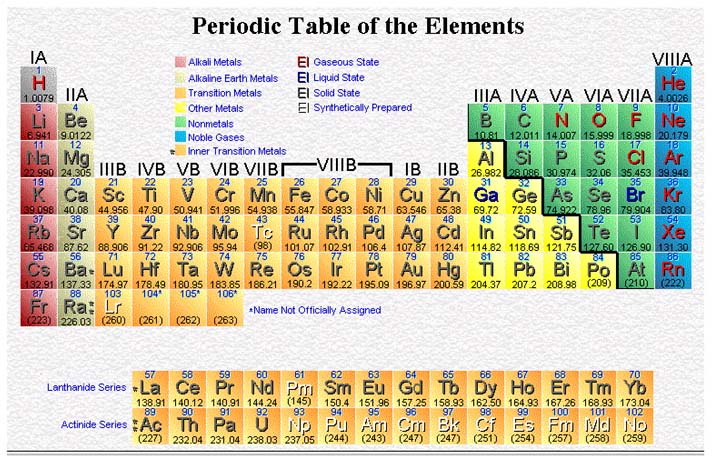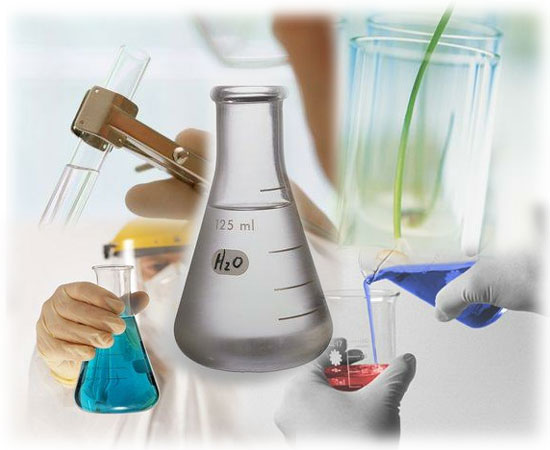CHEMISTRY Pre-AP Unit 16: Acids, Bases, and SaltsGoal: The student should be able to:

1. Give operational definitions for acids, bases, and salts.

2. Distinguish among the three acid-base theories.

3. Given the formula for an acid (base), write the formula for the conjugate base (acid).

4. Given a reaction, identify the acid, base, conjugate acid and conjugate base.

5. Explain why water is considered to be amphoteric.

6. Predict the product of a metal oxide or nonmetal oxide dissolved in water.

7. Relate the strength of an acid or base to its degree of dissociation. (K a or K b)

8. Given the molarity and ionization constant of an acid, predict its strength by calculating the percent ionization for the compound.

9. Describe the ionization steps of a polyprotic acid.

10. Describe K w and know the value of K w.

11. Using the value for K w, calculate either the hydrogen ion concentration or hydroxide ion concentration when the other is known.

12. Define pH and pOH and recognize that they are logarithmic scales.

13. Establish the relative acidity or basicity of a substance when given the pH or pOH.

14. Given the hydrogen ion concentration or hydroxide concentration, calculate the pH and/or pOH of a substance and vice versa.

15. Given the concentration of an acid or base determine the pH or pOH of the solution.

16. Explain what the function of an indicator is and how pH may be determined with indicators.

17. Describe what effect a common ion has upon the equilibrium of a reaction and upon the dissociation of a dissolved substance.

18. Explain what the function of a buffer is.

19. Define hydrolysis.

20. Given the relative strength of the parent acid and base, predict whether the water solution of the salt will be acidic, basic or neutral.

21. Explain how the process of titration is used to determine the concentration of a substance.

Activities:

April 6: Discuss Unit XV Test, Lecture Operational Definitions of acids, Bases, and Salts

April 7: Lecture Acid-Base Theories

April 10: Lecture Relative Strengths Ka, Kb, and Kw

April 11: Lecture pH and pOH, Indicators, Buffers

April 12: Lecture Common Ion Effect, Hydrolysis, Titration

April 13: Review for Unit XVI Test

April 17: Unit XVI Test

April 21: Lab: Titration

Homework:

April 7: Begin Chapter 19 Study Guide

April 10: Practice Problems p605, 606, and 609

April 11: Practice Problems p611, 612, 614, and 616

April 12: Worksheet Acids and Bases

April 13: Study for exam

April 17: Prelab Titration

April 21: Lab report due April 27

Lecture & Lab Materials (uploaded once per week):

Acid Base Theories

Relative Strenths; Ka, Kb, and Kw